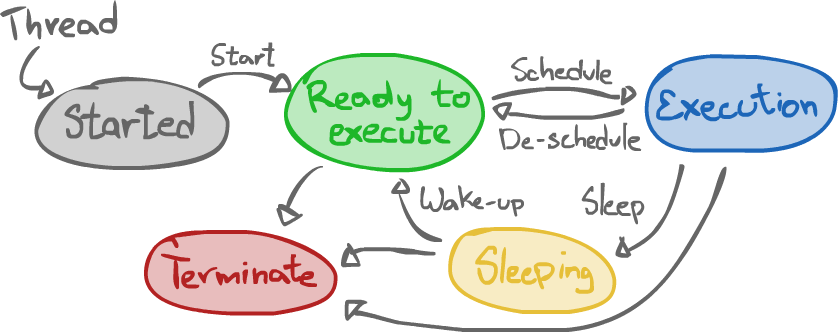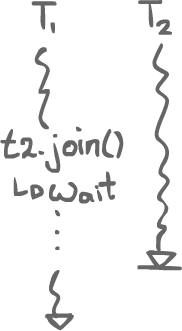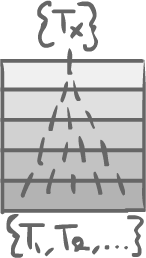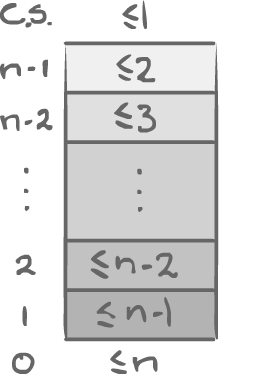# Wednesday, September 18, 2013Concurrency with Java and PThreads & Locks

## 1Java Concurrency¶

In Java, concurrency is built-in. Java was designed to support concurrency from the start and supports a rich API: java.util.concurrent.

Concurrency in Java follows an object oriented model.

Once a Thread object is instantiated, it may be started using Thread.start().

The code run by the thread is specified by myThread.run(). It either comes from overriding it when extending the class, or by extending the Thread class or implementing the Runnable interface.

The former method is typically used for using up the hierarchy, or changing the behavior of a Thread.

For generic MT execution, however, it is preferable to simply construct a Thread with a Runnable.

### 1.2When does the application end?¶

The main thread finishes when the main() function finishes. Individual threads, however, finish when their run() ends. This might happen after the end of main().

As for the whole application, it finishes when all non-daemon threads are finished.

A thread meant to be used as a service provider
The default type of threads, they are meant to be able to control the flow of the program.

### 1.3Scheduling¶There is a "nominally" priority-preemptive execution model that we may expect to be respected.

High A $\rightarrow$ B $\rightarrow$ C
Premptive D $\rightarrow$ E $\rightarrow$ F $\rightarrow$ G
Low H $\rightarrow$ I

Under this model, the OS causes high-priority threads to execute only if no higher priority thread is able to execute and threads in the next priority class get a chance to execute.

However, there is no guarantee that this model is always respected!

• There may be "run-to-completion" where there is no time-slicing. As such, threads must momentarilly give up time slice themselves using yield().
• Priorities may not be respected.
• Round-robin might not be respected. Threads of a process may execute among those of another process.

### 1.4Overview of the Thread API¶

Thread.yield()
A static method used to notify the OS that the current thread is willing to give up the CPU for a while, thus letting the thread scheduler select another thread to run.
Thread.sleep(long time)
A static method to let the current thread sleep for a certain amount of time in milliseconds. The time specified merely acts a lower-bound, and there is no guarantee on the precision of the timing.
Thread.currentThread()
A static method to "know who the thread is".
Thread.join()
A method called on a Thread to make the thread executing the call wait for this other thread to finish before going on.Thread.isAlive() (or rather wasAlive())
A method to tell if a thread is alive (started and not dead yet). In practice, it is possible for the method to return true, but for the specific thread to have died before the caller could use the value. stale value.
Thread.stop() (deprecated)
A method which forces a thread to stop executing. It is dangerous to use it because it may jeopardize the rest of the application. All the resources the thread had locked are suddenly unlocked, which may cause problems if they were in an inconsistent state.
Thread.destroy() (deprecated)
A method a bit like Thread.stop(), but without even releasing the locks. Nobody even bothered implementing that.

### 1.5Synchronization¶

Java offers basic synchronization mechanisms through the use of the synchronized and volatile keywords.

#### 1.5.1The "synchronized" keyword¶

The synchronized keyword allows an object to be locked for as long as a thread is in a defined critical section.

There are two ways to use this keyword. Firstly, around the object:

synchronized(foo) {  // foo is now locked
/* Critical section */
} // foo is now unlocked.


Say a thread executes this code. Then, for as long as it is in the critical section, any other thread will have to wait before using methods of the locked object.

Secondly, the keyword may be used when declaring a method:

synchronized void bar() { // This object is now locked
/* Critical section */
} // This object is now unlocked


This is similar to declaring the method without the synchronized keyword and having a synchronized(this) {} block inside instead. As for a static synchronized method, it is similar to having a synchronized(NameOfThatClass.class) {} block instead.

Recursive entry is allowed with synchronized methods. Even if a thread already has a lock, it can reacquire it, but it must release it as many times it has acquired it.

#### 1.5.2The "volatile" keyword¶

The volatile keyword informs the compiler that a specific variable may change asynchonously.

For example, consider that a static variable x is accessible by two threads:

• The first thread uses it in a while loop to achieve a busy loop: while (x == 0);.
• The second thread changes the value of x at some point to signal the first thread to go on.

The compiler, seeing that, x in the first thread cannot be written before the loop, may transform the loop into while (true); to accessing x at all. There was no way for it to know it would be changed by another thread.

The solution is to use the volatile keyword when declaring x, thus forbidding the compiler from optimizing the code in such a way.

#### 1.5.3Important point¶

All shared variables must be protected by synchronization, with a synchronized block or the use of the volatile keyword.

PThreads used to be the de-facto way to implement multithreading on Unix-compatible systems. Since it is a library, it can be linked with anything.

pthread_create(pthread_t *thread_handle, const pthread_attr_t *attr, void *(*start_routine) (void *), void *args);
A function to create a thread.
*thread_handle points to a buffer. The function will store the thread ID inside.
*attr points to a struct containing the thread's attributes.
*start_routine points to a function the thread will execute (like Thread.run() in Java, but with arguments)
*args points to the arguments of the function.

#### 2.1.1Attributes¶

• With the attributes, it is possible to declare a thread as DETACHED in which case we may not call join().
• By default, it is JOINABLE instead, in which case we actually must use join(). Otherwise, resources that would have been freed with join() would not be freed and resource exhaustion might happen.

### 2.2Scheduling model¶

It is possible to change the scheduling policy of a thread using the method pthread_setschedparam(pthread_t thread, int policy, const struct sched_param *param).

SCHED_RR
Round-robin, time-sliced.
SCHED_FIFO
Run-to-completion.
SCHED_OTHER
Whatever else is available.

### 2.3Mutual exclusion¶

You can define a mutex, a 1-bit lock, to achieve synchronization mechanisms.

pthread_mutex_init(pthread_mutex_t *mutex, const pthread_mutexattr_t *attr)
A function to initialize a given mutex after it has been declared.
pthread_mutex_lock(pthread_mutex_t *mutex)
A function to lock a mutex by the current thread if no other thread has the lock.
pthread_mutex_unlock(pthread_mutex_t *mutex)
A function to unlock a mutex, thus letting any other thread lock it.

In general, the same thread should lock and unlock a mutex. Otherwise, there may be some unforeseen consequences.

Mutexes cannot (by default) be locked recursively. However, multiple vendors have created "vendor extensions" for PThreads which serve to make a programmer's life easier by adding features PThreads does not originally support.

## 3Locks¶

The Peterson 2 process tie-breaker algorithm only worked with 2 processes. How could we generalize that?

### 3.1Filter Algorithm¶

The filter algorithm generalizes the algorithm to work with $n$ threads.There are $n$ levels where threads can wait before the critical section. For each level, the threads are considered pair-by-pair, until there can be only one in the critical section.There are two properties to consider:

1. If more than 1 thread tries to get into a stage, at least one is blocked.
2. At least one thread entering some stage S succeeds.
// Init
int stage[n];   // Like the flag variable, indexed by thread ID, initialized to {0, ..., 0}
int waiting[n]; // Like the turn variable, indexed by stages, initialized to {0, ..., 0}

// Enter
for (int i = 1, i < n; i++) {
stage[id] = i;
waiting[i] = id;
bool spin;
do {
spin = false;
for (int j = 0; j < n; j++) {
if (j == id) continue;
if (stage[j] >= i && waiting[i] == id) {
spin = true;
}
break;
}
} while (spin);
}

// Exit
stage[id] = 0;


## 4Correctness of the filter-lock algorithm¶

lemma:

There are at most $n-i$ threads at level $i$. ($0 \leq i \leq n-1$)

(a.k.a there will always be one thread not stuck).

### 4.1Proof by induction¶

#### 4.1.1Base Case¶

At $i = 0$, we have $\leq n$ threads, which is at most $n-i = n$. Trivial.

#### 4.1.2Inductive step¶

Induction hypothesis: At level $i-1$, we have at most $n-(i-1) = n-i+1$ threads.

At level $i$, we can use a proof by contradiction: Let's assume there are too many threads at this level. That is, $n-i+1$ threads at level $i$.

Let TA be the last threads to set waiting[i] = A.

For any other thread (at level $i$) TX, it is setting waiting[i] = X, preceding waiting[i] = A.

We know that all TX set stage[x] = i before setting waiting[i] = X.

stage[x] = i $\rightarrow$ waiting[i] = x $\rightarrow$ waiting[i] = A.

TA reads stage[x] after it sets waiting[i] = A, once it starts inspecting the other threads.

stage[x] = i $\rightarrow$ waiting[i] = x $\rightarrow$ waiting[i] = A $\rightarrow$ read_A(stage[x]).

So TA must have checked stage[x], it must have found it was greater or equal to $i$. We know it's the last one to set waiting[i] = A, so that means TA is necessarily still spinning, which is a contradiction!

Q.E.D.

We can also prove that this is starvation-free.

## 5Hardware support for mutual exclusion¶

We've seen a few algorithms for doing mutual exclusion. These algorithms work, but they're quite a bit of code to run. Those using a turn array also impose a constant number of threads constraint. To make it easier, the hardware will typically provide primitives for mutual exclusion.

### 5.1Test-and-set instruction¶

This instruction basically does two things at once. It can be used for doing a very basic spin-lock.

int test_and_set(int &x, int y) {
int temp = x;
x = y;
return temp;
} // The function is atomic!


Despite the name, it is not a test in itself, but it provides the ability for the programmer to do a test.

#### 5.1.1Spin lock based with Test-and-set¶

// Init
int lock = 0;
// Enter
while (test_and_set(lock, 1) == 1); // Spin
// Exit
lock = 0


Basically, the thread will try to set the lock to 1. Since test_and_set is atomic, if the value of lock was 0, then the thread was the first to set it to 1, and thus acquired the lock. Otherwise, it means another threads has acquired it before, so the thread should spin.

It is much easier, there is less code, but it does not have the same nice properties as the other algorithms had; instead of respecting the order of the threads, only the "luckiest" thread is the one to run.

#### 5.1.2Test-and-test-and-set¶

The spin lock with the test_and_lock keeps writing to the value during its test. This makes the algorithm quite a bit expensive. A slight variation of the usage of test_and_lock is to add a layer of testing around it. This allows to read only, and only write when it should.

delay = 1;
while (true) {
while (lock);
if (TS(lock, 1) == 0) {
break;
} else {
sleep(delay);
delay *= 2; // exponential back-off (a variation)
}
}


test_and_set only sets something, but maybe one would want to atomically increment and find out what the old value was (e.g. for the Ticket Algorithm). That's what fetch_and_add does.

int fetch_and_add(int &v, int c) {
int temp = v;
v = v + c;
return temp;
} // All atomic


Building a spin lock with it is possible, but it is left as an exercise (look out for overflow).

### 5.3Compare-and-swap¶

People quickly found there's actually a better way of doing things. Most hardware nowadays will have something usually called compare_and_swap. It is slightly more powerful: it is going to set a variable to something, but only if its former value is equal to something else.

// Set x to b if x == a
int compare_and_swap(int &x, int a, int b) {
if (x != a) return false;

x = b;
return true;
} // Atomic


Building a spin lock with it is possible as well, but again, it is left as an exercise.